## Many elementary school students in a school district currently have ear infections. A random sample of children in two different schools fou

Question

Many elementary school students in a school district currently have ear infections. A random sample of children in two different schools found that 16 of 40 at one school and 13 of 30 at the other had this infection. Conduct a test to answer if there is sufficient evidence to conclude that a difference exists between the proportion of students who have ear infections at one school and the other. Find the test statistic. [Suggestion: try to use a TI 83 or a similar calculator.]

in progress 0
7 months 2021-07-28T03:01:59+00:00 1 Answers 10 views 0

The test statistic is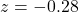Step-by-step explanation:

First, before finding the test statistic, we need to understand the central limit theorem and difference between normal variables.

Central Limit Theorem

The Central Limit Theorem estabilishes that, for a normally distributed random variable X, with mean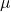and standard deviation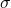, the sampling distribution of the sample means with size n can be approximated to a normal distribution with meanand standard deviation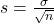.

For a skewed variable, the Central Limit Theorem can also be applied, as long as n is at least 30.

For a proportion p in a sample of size n, the sampling distribution of the sample proportion will be approximately normal with mean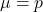and standard deviation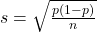Subtraction between normal variables:

When two normal variables are subtracted, the mean is the difference of the means, while the standard deviation is the square root of the sum of the variances.

A random sample of children in two different schools found that 16 of 40 at one school

This means that: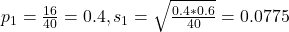13 of 30 at the other had this infection.

This means that: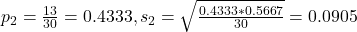Conduct a test to answer if there is sufficient evidence to conclude that a difference exists between the proportion of students who have ear infections at one school and the other.

At the null hypothesis, we test if there is no difference, that is: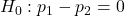And at the alternate hypothesis, we test if there is difference, that is: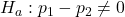The test statistic is: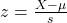In which X is the sample mean,is the value tested at the null hypothesis and s is the standard error

0 is tested at the null hypothesis:

This means that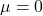From the two samples: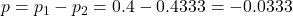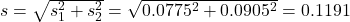Value of the test statistic: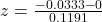The test statistic is